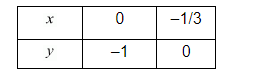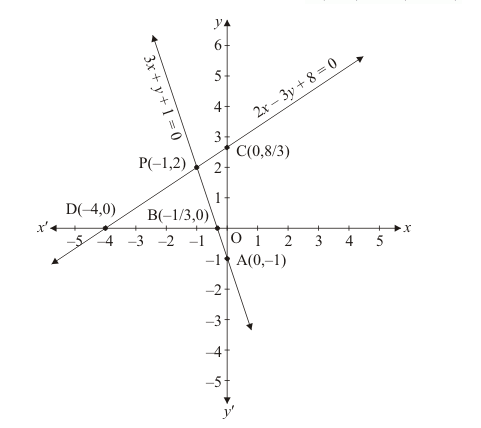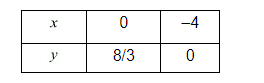Deepak Scored 45->99%ile with Bounce Back Crack Course. You can do it too!

# Solve the following systems of equations graphically:

Question:

Solve the following systems of equations graphically:

$3 x+y+1=0$

$2 x-3 y+8=0$

Solution:

The given equations are

$3 x+y+1=0 \quad \ldots \ldots .(i)$

$2 x-3 y+8=0 \quad \ldots \ldots \ldots($ ii $)$

Putting $x=0$ in equation $(i)$, we get:

$\Rightarrow 3 \times 0+y=-1$

$\Rightarrow y=-1$

$x=0, \quad y=-1$

Putting $y=0$ in equation $(i,$, we get:

$\Rightarrow 3 x+0=-1$

$\Rightarrow x=-1 / 3$

$x=-1 / 3, \quad y=0$

Use the following table to draw the graph.Draw the graph by plotting the two points $A(0,-1)$ and $B(-1 / 3,0)$ from table.Graph of the equation. (ii):

$2 x-3 y=-8 \quad$......(ii)

Putting $x=0$ in equation (ii) we ge

$\Rightarrow 2 \times 0-3 y=-8$

$\Rightarrow y=8 / 3$

$x=0, \quad y=8 / 3$

Putting $y=0$ in equation $(i i)$, we get

$\Rightarrow 2 x-3 \times 0=-8$

$\Rightarrow x=-4$

$x=-4, \quad y=0$

Use the following table to draw the graph.Draw the graph by plotting the two points $C(0,8 / 3)$ and $D(-4,0)$ from table.

The two lines intersect at points $\mathrm{P}(-1,2)$.

Hence $x=-1, \quad y=2$ is the solution.